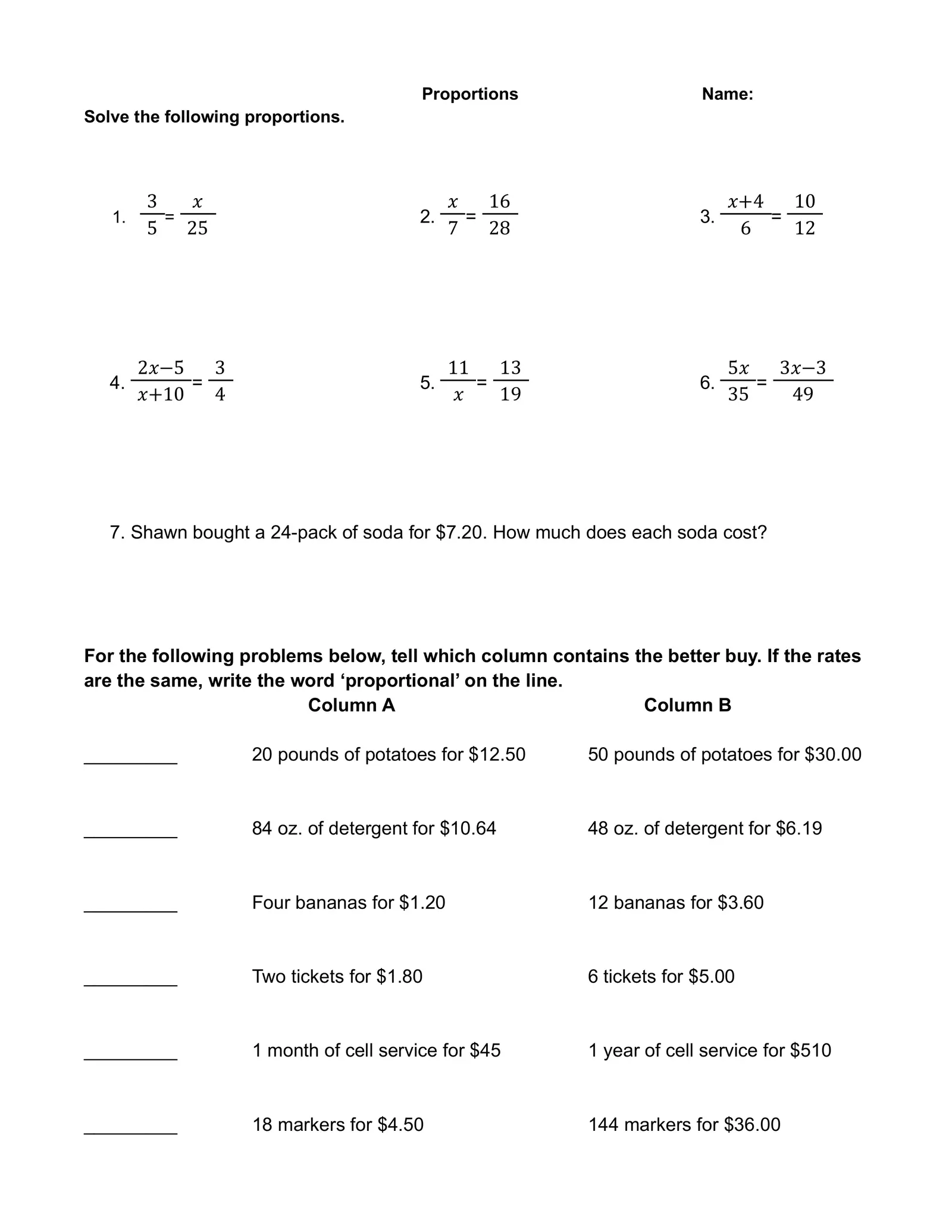# Proportions Worksheet

Created

Proportions Name: Solve the following proportions. 3 ???? ???? 16 ????+4 10 1. = 2. = 3. = 5 25 7 28 6 12 x = 15 x=4 x=1 2????−5 3 11 13 5???? 3????−3 4. = 5. = 6. = ????+10 4 ???? 19 35 49 209 1 3 x = 10 x= 13 or 16 13 x=− 4 or -0.75 or 16.08 7. Shawn bought a 24-pack of soda for \$7.20. How much does each soda cost? \$0.30 per can For the following problems below, tell which column contains the better buy. If the rates are the same, write the word ‘proportional’ on the line. Column A Column B Column B 20 pounds of potatoes for \$12.50 50 pounds of potatoes for \$30.00 Column A 84 oz. of detergent for \$10.64 48 oz. of detergent for \$6.19 Proportional Four bananas for \$1.20 12 bananas for \$3.60 Column B Two tickets for \$1.80 6 tickets for \$5.00 Column B 1 month of cell service for \$45 1 year of cell service for \$510Choose the correct operation to be performed.
Enter your answer in the space below